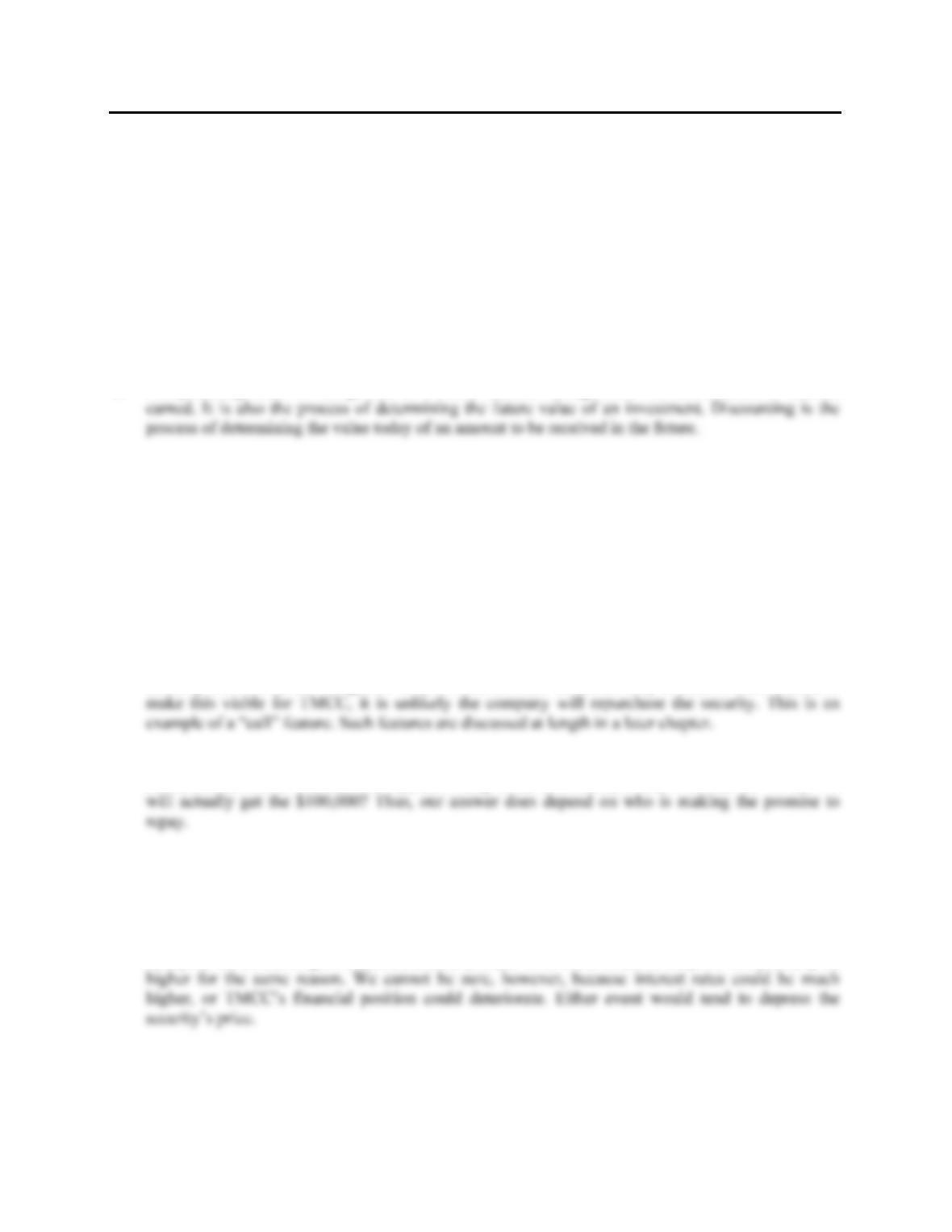Type
Quiz
Book Title
Fundamentals of Corporate Finance Standard Edition 9th Edition
ISBN 13
978-0073382395

### 978-0073382395 Chapter 5 Concepts Review and Critical Thinking Questions

April 3, 2019CHAPTER 5
INTRODUCTION TO VALUATION: THE
TIME VALUE OF MONEY
Answers to Concepts Review and Critical Thinking Questions
1. The four parts are the present value (PV), the future value (FV), the discount rate (r), and the life of
the investment (t).
2. Compounding refers to the growth of a dollar amount through time via reinvestment of interest
3. Future values grow (assuming a positive rate of return); present values shrink.
4. The future value rises (assuming it’s positive); the present value falls.
5. It would appear to be both deceptive and unethical to run such an ad without a disclaimer or
explanation.
6. It’s a reflection of the time value of money. TMCC gets to use the \$24,099. If TMCC uses it wisely,
it will be worth more than \$100,000 in thirty years.
7. This will probably make the security less desirable. TMCC will only repurchase the security prior to
maturity if it is to its advantage, i.e. interest rates decline. Given the drop in interest rates needed to
8. The key considerations would be: (1) Is the rate of return implicit in the offer attractive relative to
other, similar risk investments? and (2) How risky is the investment; i.e., how certain are we that we
9. The Treasury security would have a somewhat higher price because the Treasury is the strongest of
all borrowers.
10. The price would be higher because, as time passes, the price of the security will tend to rise toward
\$100,000. This rise is just a reflection of the time value of money. As time passes, the time until
receipt of the \$100,000 grows shorter, and the present value rises. In 2019, the price will probably be